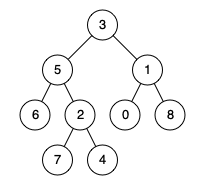AI 数据科学认证 -2021 年的最佳选择 John 易筋 ARTS 打卡 Week 44John(易筋)

236. Lowest Common Ancestor of a Binary Tree

Given a binary tree, find the lowest common ancestor (LCA) of two given nodes in the tree.

According to the definition of LCA on Wikipedia: “The lowest common ancestor is defined between two nodes p and q as the lowest node in T that has both p and q as descendants (where we allow a node to be a descendant of itself).”

Example 1:Input: root = [3,5,1,6,2,0,8,null,null,7,4], p = 5, q = 1Output: 3Explanation: The LCA of nodes 5 and 1 is 3.

Example 2:Input: root = [3,5,1,6,2,0,8,null,null,7,4], p = 5, q = 4Output: 5Explanation: The LCA of nodes 5 and 4 is 5, since a node can be a descendant of itself according to the LCA definition.

Example 3:

Input: root = [1,2], p = 1, q = 2Output: 1

Constraints:

The number of nodes in the tree is in the range [2, 105].-109 <= Node.val <= 109All Node.val are unique.p != qp and q will exist in the tree.

dfs 深度优先解法

1. 用深度优先算法，如果没有找到，那么返回 null。

2. 两边都找到，那么当前节点就是结果；

3. 如果 left 子树为空，那么肯定在右子树里面；

4. 如果 right 子树为空，那么可定在左子树里面。

/** * Definition for a binary tree node. * public class TreeNode { *     int val; *     TreeNode left; *     TreeNode right; *     TreeNode(int x) { val = x; } * } */class Solution {    public TreeNode lowestCommonAncestor(TreeNode root, TreeNode p, TreeNode q) {        if (root == null || root == p || root == q) return root;        TreeNode left = lowestCommonAncestor(root.left, p, q);        TreeNode right = lowestCommonAncestor(root.right, p, q);        if (left != null && right != null) return root;        return left == null ? right : left;    }}

遍历解法

1. 把所有节点跟父节点的关系存储到 parent map 中；

2. stack 仅仅为为了 bfs 广度优先搜索的必要。

3. 把 p 自己，p 的所有父节点，祖父节点，一直到 root 都放到 ancestors；

4. ancestors 如果包含 q，说明同时是 p，q 的公共祖先；否则就找 q 的上一个父节点，依次循环，直到在 ancestors 中找到。

public class Solution {    public TreeNode lowestCommonAncestor(TreeNode root, TreeNode p, TreeNode q) {        Map<TreeNode, TreeNode> parent = new HashMap<>();        Deque<TreeNode> stack = new ArrayDeque<>();        parent.put(root, null);        stack.push(root);
while (!parent.containsKey(p) || !parent.containsKey(q)) { TreeNode node = stack.pop(); if (node.left != null) { parent.put(node.left, node); stack.push(node.left); } if (node.right != null) { parent.put(node.right, node); stack.push(node.right); } } Set<TreeNode> ancestors = new HashSet<>(); while (p != null) { ancestors.add(p); p = parent.get(p); } while (!ancestors.contains(q)) q = parent.get(q); return q; }}

数据科学家做什么工作？

🔗数据科学专业人员的工作在银行，金融，教育和医疗保健等领域得到广泛使用也就不足为奇了。而且，对数据的日益关注导致对诸如人工智能和机器学习之类的相关技能的需求增加。

数据科学专业人员能赚多少薪水？

👉入门级：这是指应届毕业生或经验丰富的专业人员从不同领域转换而没有相关工作经验。他们倾向于更多地学习和实践新技能，因此他们从雇主那里接受了适当的培训。年薪估计为 69,000 美元。

👉中级：年薪中位数为 89,000 美元。

👉经验丰富：这些专业人员在数据科学事业中拥有丰富的经验。他们的年薪中位数为 1,28,000 美元。

manager 在经理级别有经验：年薪中位数可能高达 184,000 美元。

就位置而言，以下是估计的年薪：

·印度：708,012 印度卢比（约合 9,507 美元，来源：PayScale）·在美国：96,106 美元（来源：PayScale）

以下是获得最佳数据科学认证的优势：

·突出投资组合和简历的其他技能·改善工作前景·访问职业频道，招聘实验室和雇主评论·促进职业道路之间的过渡换句话说，认证可以极大地促进数据科学事业的发展。雇主非常看重认证，这让工作绩效和技能集可以为员工说话。他们还表明，该人愿意付出额外的努力来提高他或她的技能。

2.数据科学专业（约翰霍普金斯大学和 Coursera）

3.高级大数据分析师（美国数据科学理事会— DASCA）对于寻求加速大数据分析增长的专业人士而言，高级大数据分析师（或称 SBDA™）是世界上最可信的第三方独立于厂商的认证。它是国际上公认和接受的，主要针对大数据或市场研究专业人员。它涵盖以下内容：·数据科学的基础：Python 和 R·数据科学数学：数学和统计学中的重要概念·处理数据：使用 Python 和 R 管理海量数据·进行数据分析：使用 Hadoop 生态系统分析大数据·数据可视化：Tableau 可视化并显示结果·机器学习和 AI 中的大数据：在 ML 和 AI 应用程序中使用 R 和 Python·业务实施：在业务级数据分析中使用 R 和 Python·实施分析：MongoDB，消息传递，企业搜索，云和集群⏲持续时间：6 个月here在这里注册！

说明

swift 5 提出 PropertyWrapper，类似于 AOP 拦截器的思想，在属性的更改之前，做个拦截处理，以下为官网资料的翻译

PropertyWrapper

@propertyWrapperstruct TwelveOrLess {    private var number: Int    init() { self.number = 0 }    var wrappedValue: Int {        get { return number }        set { number = min(newValue, 12) }    }}

struct SmallRectangle {    @TwelveOrLess var height: Int    @TwelveOrLess var width: Int}
var rectangle = SmallRectangle()print(rectangle.height)// Prints "0"
rectangle.height = 10print(rectangle.height)// Prints "10"
rectangle.height = 24print(rectangle.height)// Prints "12"

struct SmallRectangle {    private var _height = TwelveOrLess()    private var _width = TwelveOrLess()    var height: Int {        get { return _height.wrappedValue }        set { _height.wrappedValue = newValue }    }    var width: Int {        get { return _width.wrappedValue }        set { _width.wrappedValue = newValue }    }}

@propertyWrapperstruct SmallNumber {    private var maximum: Int    private var number: Int
var wrappedValue: Int { get { return number } set { number = min(newValue, maximum) } }
init() { maximum = 12 number = 0 } init(wrappedValue: Int) { maximum = 12 number = min(wrappedValue, maximum) } init(wrappedValue: Int, maximum: Int) { self.maximum = maximum number = min(wrappedValue, maximum) }}

struct ZeroRectangle {    @SmallNumber var height: Int    @SmallNumber var width: Int}
var zeroRectangle = ZeroRectangle()print(zeroRectangle.height, zeroRectangle.width)// Prints "0 0"

struct UnitRectangle {    @SmallNumber var height: Int = 1    @SmallNumber var width: Int = 1}
var unitRectangle = UnitRectangle()print(unitRectangle.height, unitRectangle.width)// Prints "1 1"

struct NarrowRectangle {    @SmallNumber(wrappedValue: 2, maximum: 5) var height: Int    @SmallNumber(wrappedValue: 3, maximum: 4) var width: Int}
var narrowRectangle = NarrowRectangle()print(narrowRectangle.height, narrowRectangle.width)// Prints "2 3"
narrowRectangle.height = 100narrowRectangle.width = 100print(narrowRectangle.height, narrowRectangle.width)// Prints "5 4"

struct MixedRectangle {    @SmallNumber var height: Int = 1    @SmallNumber(maximum: 9) var width: Int = 2}
var mixedRectangle = MixedRectangle()print(mixedRectangle.height)// Prints "1"
mixedRectangle.height = 20print(mixedRectangle.height)// Prints "12"

SmallNumber 包装的实例 height 是通过调用来创建的，该实例使用默认的最大值 12。包装的实例是通过调用来创建的。SmallNumber(wrappedValue: 1)widthSmallNumber(wrappedValue: 2, maximum: 9)

@propertyWrapperstruct SmallNumber {    private var number: Int    var projectedValue: Bool    init() {        self.number = 0        self.projectedValue = false    }    var wrappedValue: Int {        get { return number }        set {            if newValue > 12 {                number = 12                projectedValue = true            } else {                number = newValue                projectedValue = false            }        }    }}struct SomeStructure {    @SmallNumber var someNumber: Int}var someStructure = SomeStructure()
someStructure.someNumber = 4print(someStructure.$someNumber)// Prints "false" someStructure.someNumber = 55print(someStructure.$someNumber)// Prints "true"

enum Size {    case small, large}
struct SizedRectangle { @SmallNumber var height: Int @SmallNumber var width: Int
mutating func resize(to size: Size) -> Bool { switch size { case .small: height = 10 width = 20 case .large: height = 100 width = 100 } return $height ||$width }}

1. 运营是做什么的

• <font color='red'>运</font>转·经<font color='red'>营</font>. 产品经理经常拆房子、盖新房子。经营是要持续经营一个产品。

• CEO、COO(Apple 的 Tim Cook 以前就是)、CTO、CFO、CIO......

• 运营其实是个容量很大的工种

• 运营不是【打杂的】，但运营的工作中一定包含大量【事务性执行工作】。

• 如果你决定独立在社区里开个服装店......产品要租个店铺，装修，想着到哪里进货，选品的风格，摆二维码，怎么开门。运营要持续卖货，补货，经常调整一下布置，清仓等。产品生，运营养。运营负责的细水长流。

2. 运营工作的抽象套路

![在这里插入图片描述](https://img-blog.csdnimg.cn/20210407204800332.png#pic_center =500x)

3. 几种常听到的运营岗位

• 用户运营：针对用户拉新、留存，制定策略。

• 平台运营：针对平台的指标优化，GMV，客单价，一般会做跟用户相关的策略等。很像美联储、央行运用宏观的手段，达成目标。

• 产品运营：深入地影响产品的特性。

• 内容运营：买内容，写内容，发内容，传播内容。

• 社群社区运营：社群社区用户活跃。

• 新媒体运营：转发抽奖，增加粉丝量，阅读量。

• 活动运营：抽奖、发优惠券、周年庆等。

• 运营推广：通过数据手段做推广，比如快手要求运营会 SQL。做自媒体的广告，搜索引擎的广告投放。

• 渠道运营：管渠道，比如线下推广，电梯广告推广，流媒体平台的推广。

• 店铺运营：订单管理，发货，上新，活动等。

3.1 用户运营

• 目标：拉新、促活、留存、传播、收入。

• 常见的运营手段：☞ 给用户分出不同的类。给用户打标签。☞ 跟用户接触上并有效地传递一些信息给用户☞ 引导用户知道一些事情☞ 或跟用户做一些【交易】，让用户行动☞ 看看效果，调整策略，继续跟用户【沟通】。

• 知道用户什么习性

• 能跟用户说上话

• 说的话用户能听进去

• 听进去了能行动

• 以上过程能持续

3.2 社区运营

• 目标：更多关系、更多内容、更强吸引力。社区跟人数成正比，跟人数的关系的平方成正比。

• 常见的运营手段（以内容为例）☞ 设置排行榜，奖励发内容的☞ 定点邀请发内容☞ 内容的推荐引导，内容加精华，加分。☞ 建立例行内容机制☞ 自己硬发

4. 产品经理与运营的关系与配合

• 生孩子 & 养孩子。

• 紧贴运营，用运营的直觉触感进行产品迭代的尺子。春江水暖鸭先知，产品好与不好，客服和运营知道比较早。客户会投诉，运营比如发优惠券转换很高，那么可以推新用户立减。

• 将运营规则产品化，而不是只做产品 & 运营工具。

5. 运营工作给产品工作的启发

• 用运营的思路做产品：比如微信

• 不断尝试和细微修正迭代的小循环 & 基于整体目标、策略和资源的大循环。

• 有高视角，也有低视角，仰手接飞猱(nao)，俯身散马蹄。

• 以【活】的目标和姿态去【生】，从动态运转的角度反思静态设计。

• 设计和开发的不是一个架子（空中楼阁），而是一个能够流动的体系。

• 细节的雕琢能力、文案能力、极强的执行力。评论